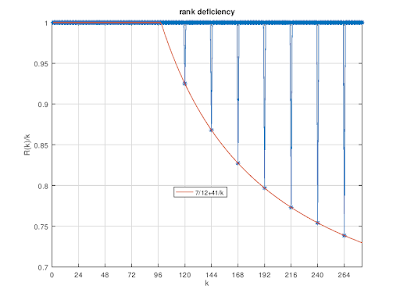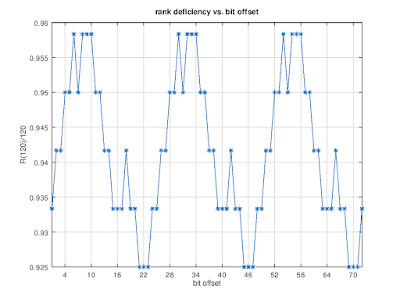## Tuesday, June 25, 2019

### 4800 symb/sec QPSK signal on 10160 kHz (2)

This in an update related to the last blog post. As before, all analysis is done in GNU octave.

In order to extract the bit stream from the signal on 10160 kHz we assume that it (D)QPSK modulated and use the gray code mapping [0,1,2,3] -> [0,1,3,2].

## Assuming QPSK modulation

The extracted bits are shown below in terms of 894-bit long frames.bit stream in terms of 24×41 frames (QPSK)

The rank-deficiency analysis of this bit stream indicates the presence of a convolutional code:rank-deficiency (QPSK)

For obtaining the rank-deficiencies above, the bit stream has been aligned by using an offset for which the R(k)/k is minimal, i.e., at 10+m×24:alignment of bit stream for the rank-deficiency analysis (QPSK)

As a result, the code rate is n/k=1/4, and the length of the dual code is 41. The latter is quite large and therefore hints at the use of some form of Turbo Code, or something similar to it.

## Assuming DQPSK modulation

The extracted bits are shown below in terms of 894-bit long frames. Unlike for QPSK modulation the bits in positions [1,18,19,24]+m×24 are the same in each frame.zoom of bit stream in terms of 24×41 frames (DQPSK)

Also assuming DQPSK modulation, the presence of a convolutional code is detected; it has code rate 7/12 and the length of the dual code is 41. (This might be a Turbo Code with 14 systematic bits and 5+5 parity bits)rank deficiency (DQPSK)alignment of bit stream for the rank-deficiency analysis (DQPSK)

## Summary

It is unlikely by coincidence that the frame length is 24×41, the length of the dual code is 41, and the distance between the rank deficiencies is 24.

The fixed bits in each frame when assuming DQPSK modulation are likely being used for frame synchronization: in this case, 4 of the 14 systematic bits are used for frame alignment and there are 5+5 parity bits.

Obviously it would be interesting to figure out the full parameters of the convolutional coding.Anonymous said...

A recent ITU BR IFIC Publication

https://www.itu.int/en/ITU-R/terrestrial/brific/BRIFIC/Article11-Index/ific2896.pdf

Shows

9.3400 MTXC JUNGLINSTER LUX 6°E15'52'' 49°N43'07'' FX
10.1600 MTXC JUNGLINSTER LUX 6°E15'52'' 49°N43'07'' FX

This indicates that it is a Fixed Link service and not a Broadcast transmission.

These co-ordinates are very close (maybe a conversion error ?) to the former Radio Luxembourg LW BC site at Junglinster which also used to transmit DRM on very similar frequencies and has a suitable antenna in place.

https://www.bce.lu/transmitters/

https://goo.gl/maps/waYBgD9T5h3yXzsu6

The BC site is now being developed by BCE as a data center

https://www.bce.lu/datacentre/

As Luxembourg is an important financial trading and cryptocurrency exchange, my guess is that the transmissions may be associated with High Frequency Financial Trading.

Martin - G8JNJ

Antonio Anselmi said...